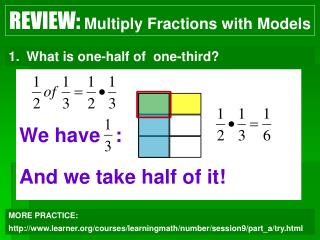DownloadDownload PresentationWe have : And we take half of it!

# We have : And we take half of it!

Télécharger la présentation## We have : And we take half of it!

- - - - - - - - - - - - - - - - - - - - - - - - - - - E N D - - - - - - - - - - - - - - - - - - - - - - - - - - -
##### Presentation Transcript

1. REVIEW: Multiply Fractions with Models What is one-half of one-third? We have : And we take half of it! MORE PRACTICE: http://www.learner.org/courses/learningmath/number/session9/part_a/try.html

2. Divide Fractions

3. NOTES: Divide Fractions with Models means how many ’s are in ? So, I have 4 groups of 2! Let’s try a fraction: • means how many ’s are in ? • So, I have 8 groups of ½!

4. NOTES: Dividing Fractions DO NOT Have to FIND COMMON DENOMINATORS! VOCAB: The product of a fraction and its reciprocal (or multiplicative inverse) is 1. …To make a reciprocal, flip the fraction upside down! Rewrite in fraction form – Turn mixed #’s & whole #’s into improper fractions! 2. Multiply by the reciprocal. **Keep It – Change It – Flip It Method 3. As always…SIMPLIFY!

5. NOTES: Dividing Fractions When Dividing Fractions Don't be Shy Flip the 2nd And Multiply!

6. EXAMPLES: Dividing Fractions 1 2 1 3 1

7. EXAMPLES: Dividing Fractions 1 4 3 1 5 19 5 6 4

8. **If you want to find common denominators, you can. Your denominators will divide away, and you will divide the numerators straight across. Once you have a common denominator, divide straight across. 3 ÷ 2 =